# 12

### Produced As Part Of The SERI ML Alignment Theory Scholars Program 2022 Research Sprint Under John Wentworth

Two Deep Neural Networks with wildly different parameters can produce equally good results. Not only can a tweak to parameters leave performance unchanged, but in many cases, two neural networks with completely different weights and biases produce identical outputs for any input.

The motivating question:
Given two optimal models in a neural network's weight space, is it possible to find a path between them comprised entirely of other optimal models?

In other words, can we find a continuous path of tweaks from the first optimal model to the second without reducing performance at any point in the process?

Ultimately, we hope that the study of equivalently optimal models would lead to advances in interpretability: for example, by producing models that are simultaneously optimal and interpretable.

# Introduction

Your friend has invited you to go hiking in the Swiss Alps. Despite extensive planning, you completely failed to clarify which mountain you were supposed to climb. So now you're both currently standing on different mountaintops Communicating via yodels, your friend requests that you hike over to the mountain he's already on; after all, he was the one who organised the trip.

How should you reach your friend? A straight line path would certainly work, but it's such a hassle. Hiking all the way back down and then up another mountain? Madness.

Looking at your map, you notice there's a long ridge that leaves your mountain at roughly the same height. Does this ridge to reach your friend's mountain? possible to follow this ridge to reach your friend's mountain?

Similarly, we can envision two optimal models in a neural net's weight space that share a "ridge" in the loss landscape. There is a natural intuition that we should be able to "walk" between these two points without leaving the ridge. Does this intuition hold up? And what of two neural networks some distance apart in the loss landscape that have both gotten close to the global minima? Can we find a way to walk from one to the other without leaving the ridge?

To begin our investigation into the structure of these "ridges" we viewed these structures as comprising the Rashomon manifold, the subset of the weight space comprised of models that minimize the loss function. In the 2018 paper, Garipov et al. demonstrated that a simple curve is often sufficient to connect two points within the Rashomon manifold.

In our research, we applied the technique of Garipov et al. to path-connect, within a shared weight space, two models that have been trained to be optimal (at an image recognition task with respect to a given dataset). Then, we collected local data along the connecting path. One such local datum is the Hessian of the loss function with respect to the parameters. Its eigendecomposition tells us which directions to travel locally if we want to leave the Rashomon manifold, as well as which directions to travel locally if we want to stay within it. We present a formal description below.

# Theory

Consider a neural net.

Let  denote the weight parameters of the trained model yielded by the neural net.

Let  denote the loss function, a real-valued function on the parameter space that the model has been trained to minimize. Assume the loss function  is "nice and smooth''; for example,  a neural net that uses skip architecture tends to produce a nice, smooth loss landscape.

We should expect the local minima of a nice loss function to be divided into connected "ridges." Formally, if the loss function is smooth and moreover a submersion, then every level set  is a manifold. In particular, the level set of optimal models  is a manifold, whose connected components are the "ridges." A manifold is a geometric shape that looks locally, but not necessarily globally, like Euclidean space (like  for some finite , which is the dimension of the manifold). An example of a manifold is a circle, which locally looks like a one-dimensional line, but not globally. A smooth manifold is a manifold without any kinks or corners. Contrast a circle, which is a smooth 1-dimensional manifold, with a star outline, which is a non-smooth 1-dimensional manifold.

Of course, the manifold comprised of optimal models  is going to be a lot more complicated and higher-dimensional. The following example is illustrative of how difficult it is in general to globally visualize manifolds, even if they are nice spaces that are locally Euclidean.

So OK, let's say for the sake of argument that the set of optimal models  is a (locally) nice manifold, even if it's difficult to visualize. What do we know about its structure?

Empirical work suggests the following pattern. When the class of models  is underparametrized (i.e., low-dimensional linear regression, small-scale neural net architecture), there can be many ridges of local minima. This means that the precise choices of the initial parameter vector and of the optimization method (e.g., gradient descent without dropout vs. with dropout) can affect the ridge that the model will fall into during training.

However, when the class of models is sufficiently overparametrized (i.e., large-scale neural net architecture), we suspect that eventually have just one connected ridge of local minima: the ridge of global minima. The dimension of this single ridge is smaller than the dimension  of the whole weight space, but still quite high-dimensional. Intuitively, the large number of linearly independent zero-loss directions at a global minimum allow for many opportunities to path-connect towards a local minimum while staying within the ridge, making all local minima globally minimal.

There are two ways to think about the ridge of global minima. The first way, common in the literature (see, for example, Semonova et al. and Section 9 here), is to consider the subset of models in the parameter space that achieve sufficiently low loss. This subset is called the Rashomon set:

Let  for a positive , where  is the global minimum. If  is sufficiently small, the Rashomon set becomes a sufficiently sparse subset of the parameter space .

We propose a second way. We consider the Rashomon set as the set of all global minimizers,

.

It is plausible that the level set  of global minimizers is a connected smooth submanifold. (This is often true in differential geometry. As long as  is a "nice" type of map called a submersion, every level set  is a smooth embedded submanifold of the domain .)

We call the submanifold  of optimal models the Rashomon manifold, distinct from "Rashomon set'" (to distinguish from the first definition). Note that the first definition is highly related to the second definition. The Rashomon set denoting the first definition,

is a "thickening" of the Rashomon manifold   denoting the second definition.

How might this help deep-learning interpretability? By knowing the data of the Rashomon manifold , we can hope to find an element in the intersection  for a subset  of interpretable models in the total model space. The neural-net model corresponding to this element would be simultaneously interpretable and optimal.

If the subset  of interpretable models is also "nice" in the differential-geometric sense (say, also a smooth submanifold of ), then the intersection  is also similarly "nice." This means that we may be able to use tools from differential geometry to probe for an element in this "nice" intersection: an example of a model that is simultaneously interpretable and optimal. These differential-geometric tools include bundles, vector fields, differential forms, parallel transport, and flows.

One potential choice of a subset of interpretable models  which is geometrically "nice'' is the submanifold of models which is prunable in a certain way. For example, the submanifold defined by the system of equations , where  are the output connections of a given neuron , is comprised of models which can be pruned by removing neuron number . Thus, we may be able to maximally prune a neural net (with respect to the given dataset) by using an algorithm of the following form:

1. Find in the Rashomon manifold an optimal model that can be pruned in some way.
2. Prune the neural net in that way.
3. Repeat Steps 1 and 2 until there are no simultaneously prunable and optimal models anymore.

It is consequently plausible that studying the Rashomon manifold will be crucial for deep-learning interpretability.

How can we study the Rashomon manifold? One way is to take the Hessian of the loss function  at a point  and find the number of zero eigenvalues: the number of linearly independent zero-loss directions when locally starting from . This number is the dimension of the Rashomon manifold.  (See Sagun et al. for an example of this approach.)

To see why this is true, let us investigate what the Hessian is, and why it is important for probing local selection pressures at an optimal model.

The Hessian of a function  is defined by the matrix of second derivatives,

.

A three-dimensional example is helpful. Consider the submanifold of  cut out by the loss function . The global mimimum of  is , and the Rashomon manifold, given by

,

is the -axis.

Choose any optimal model on this line, say . Since this point is on the Rashomon manifold, we have ; the gradient vanishes at this point . So, the dominant local effects, the dominant selection pressures, are second-order. At , a small perturbation in the direction of any vector with zero in the -entry will result in an accelerating penalty to the loss function. The acceleration rate is  when going along either the positive or the negative -direction. The acceleration rate is  when going along either the positive or the negative -direction. These directions correspond to the major and the minor axes of the ellipses you get by looking at level sets  for  small (while keeping the zero-loss direction's variable, , to be constant).

And indeed, the ellipse axes' directions and lengths are precisely captured by the Hessian matrix at ,

.

The eigenvectors  and  represent the directions of the axes of the local selection effects' parabola, and their corresponding eigenvalues  and  represent the magnitudes of the selection pressures in these axes' directions. The zero eigenvector  is the sole zero-loss direction at point : the sole direction starting from  that remains contained in the Rashomon manifold. This is because the Rashomon manifold of  is one-dimensional (indeed, it is the -axis).

The Hessian of a higher-dimensional loss function essentially behaves in the same way. The eigenvectors with positive eigenvalue serve as (hyper)ellipsoid axes that span the nonzero-loss directions. When moving in these directions, one experiences an accelerating penalty to the loss function.

The eigenvectors with zero eigenvalue span the zero-loss directions. When moving in these directions, one remains in the Rashomon set.

And since every point of the Rashomon manifold is a global minimizer, and therefore also a local minimizer, negative eigenvalues do not occur. In math terminology, the Hessian matrix is positive-semidefinite at every local minimizer.

The Hessian at a given point on the Rashomon manifold is an example of local data. The dimension of the Rashomon manifold is an example of global data. Generally, we can hope to compute sufficiently many local and/or global data of the Rashomon manifold, enough to (1) prove or disprove the nonemptiness of , and if the former is true, (2) construct one such point in . This can potentially be done via tools from differential geometry, or from the more broadly applicable tools of topology. Successfully doing so would yield a simultaneously optimal and interpretable model for the given dataset.

# Procedure

As mentioned above, we first reproduced the results of this paper on connectivity between optima, using a smaller architecture of PreResNet20 (~270k params) .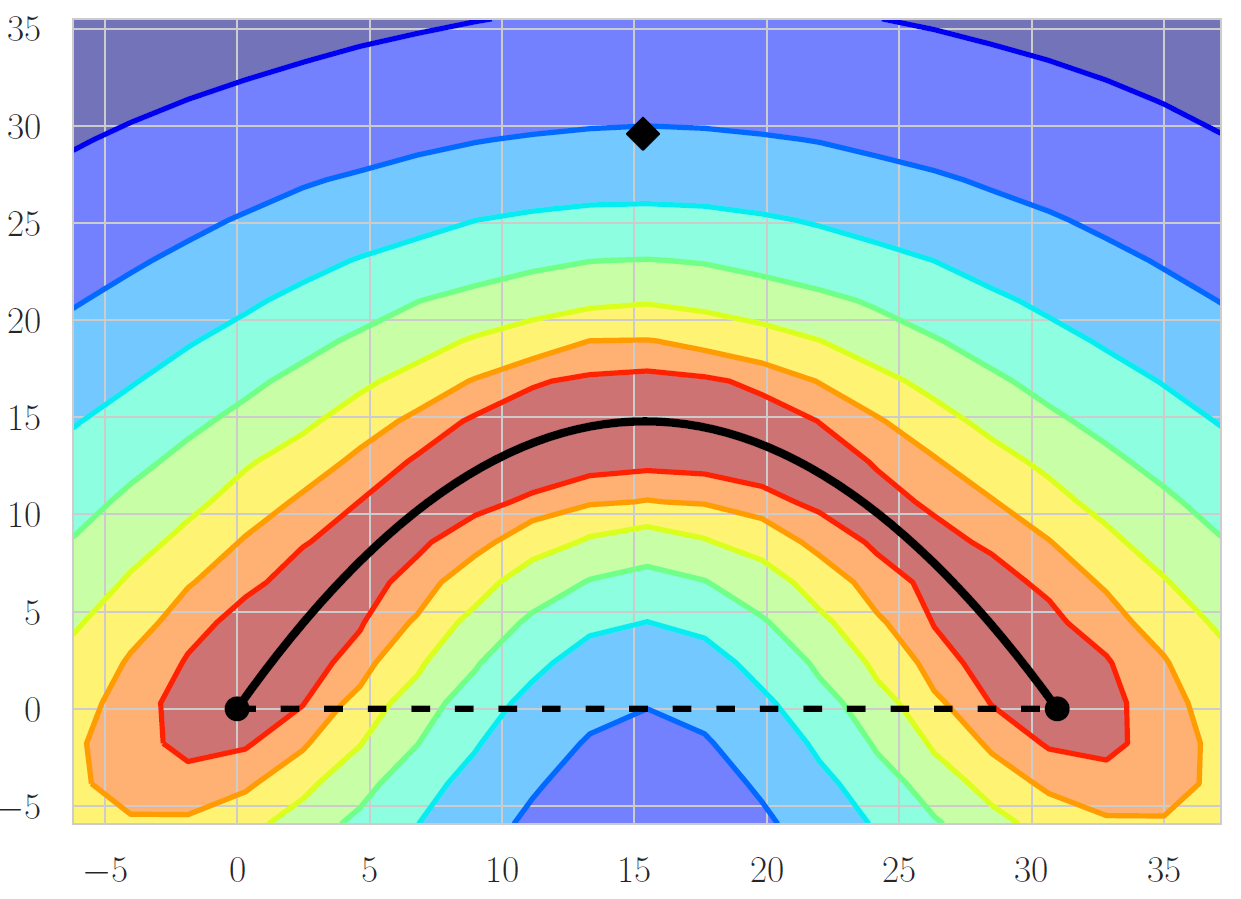This is a Bézier curve connecting 2 separately trained models along the same ridge in the loss landscape. The dashed line is a straight line path between the 2 weight vectors. The contour plot was made by calculating losses in a grid pattern.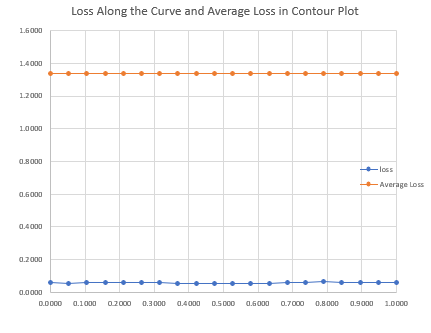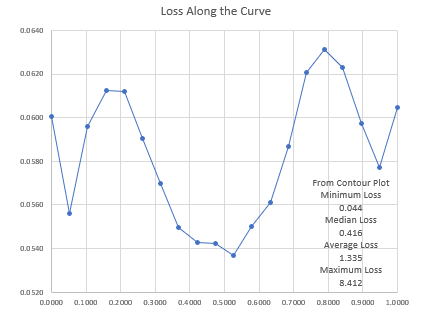For different points along this curve we have calculated.

A Jacobian of outputs with respect to inputs. Can be used for linear probes of functionality and may be able to identify how the network changes across the ridge.
A Jacobian of loss with respect to params (as expected was close to 0 norm)

The norms of the Vector Hessian Products in the straight line path direction (-axis on the graph), the detour direction (-axis on graph), and the tangent line to the curve of .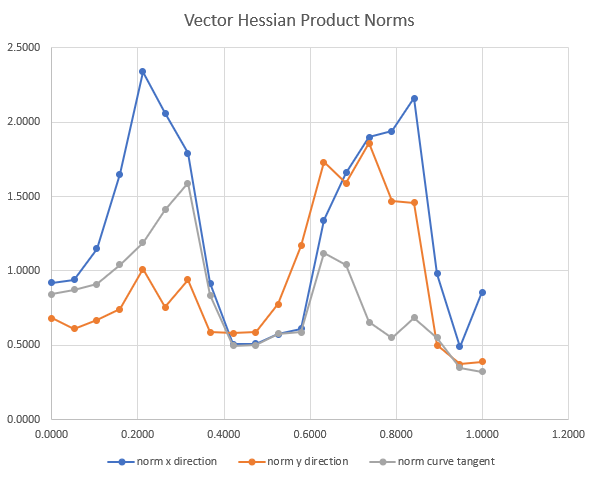# Future Pathways

Unfortunately, the nature of a sprint means we couldn't dedicate as much time to this as we would have liked. To investigate the Hessian within the Rashomon Set more closely we need significantly more computing power or a perhaps a more careful analytic approach.

We are also very keen to explore the structure of the Rashomon set. Specifically we believe much can be gleaned from exploring symmetries in the set.

• Take more Hessian vector products of models moving in the direction of the vector. Combine these values in some way to get a better estimate. The Hessian vector product on the tangent direction to the constant loss curve was smaller, but not zero like we expected and this may be due to noise.
• Use linear probes on the Jacobians of outputs with respect to inputs to identify functional similarities and differences between the models on the curve. The paper by Garipov et. al. showed that an ensemble of these models performs better than an individual one, which implies that some functional differences exist and the ridge is not simply due to irrelevant parameters.
• Jacobian of outputs with respect to parameters. Due to cuda running out of the 6gb gpu memory on my laptop.
• Using PyHessian to get top eigenvalues, trace and eigenvalue density of the Hessian of loss with respect to params. Getting a memory leak and infinite loop of params self referencing their gradients. This worked once in the colab notebook, so I have a suspicion it is a windows version bug. This is the graph that generated.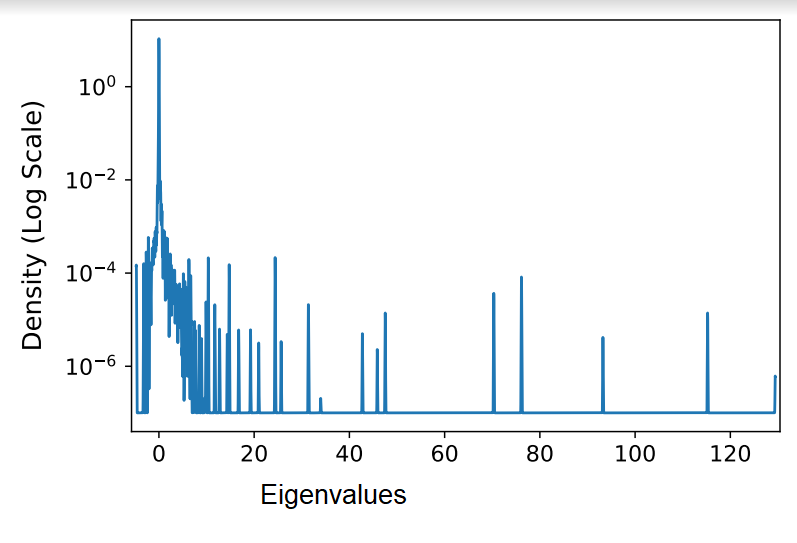• Using the density graph at different points we should be able to determine if the number of dimensions on the ridge remains constant.
• Another possibility is using the fast ensembling method of using a cyclic learning rate schedule to move along the ridge by leaving it and converging back to it in a different place. This should get models in many directions on the ridge instead of following a single curve.

New Comment